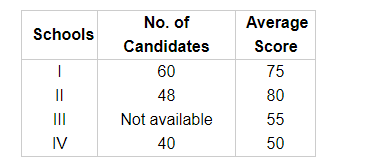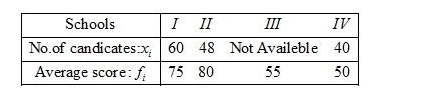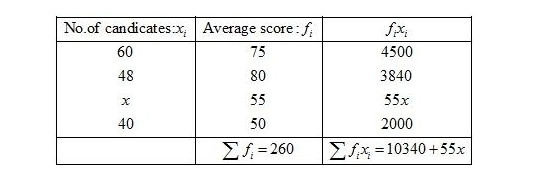# Candidate of four schools appear in a mathematics test.

Question:

Candidate of four schools appear in a mathematics test. The data were as follows:If the average score of the candidates of all the four schools is 66, find the number of candidates that appeared from school III.

Solution:

Given:Mean score of the candidates $=66$

Let the number of candidates that appeared from school $I / I$ be $x$.

First of all prepare the frequency table in such a way that its first column consists of the values of the variate $\left(x_{i}\right)$ and the second column the corresponding frequencies $\left(f_{i}\right)$.

Thereafter multiply the frequency of each row with corresponding values of variable to obtain third column containing $\left(f_{i} x_{i}\right)$.

Then, sum of all entries in the column second and denoted by $\sum f_{i}$ and in the third column to obtain $\sum f_{i} x_{i}$.We know that mean, $\bar{X}=\frac{\sum f_{i} x_{i}}{\sum f_{i}}$

$66=\frac{10340+55 x}{260}$

By using cross multiplication method,

$10340+55 x=17160$

$55 x=17160-10340$

$x=\frac{6820}{55}$

$=124$

Hence, the number of candidates that appeared from school III is 124.